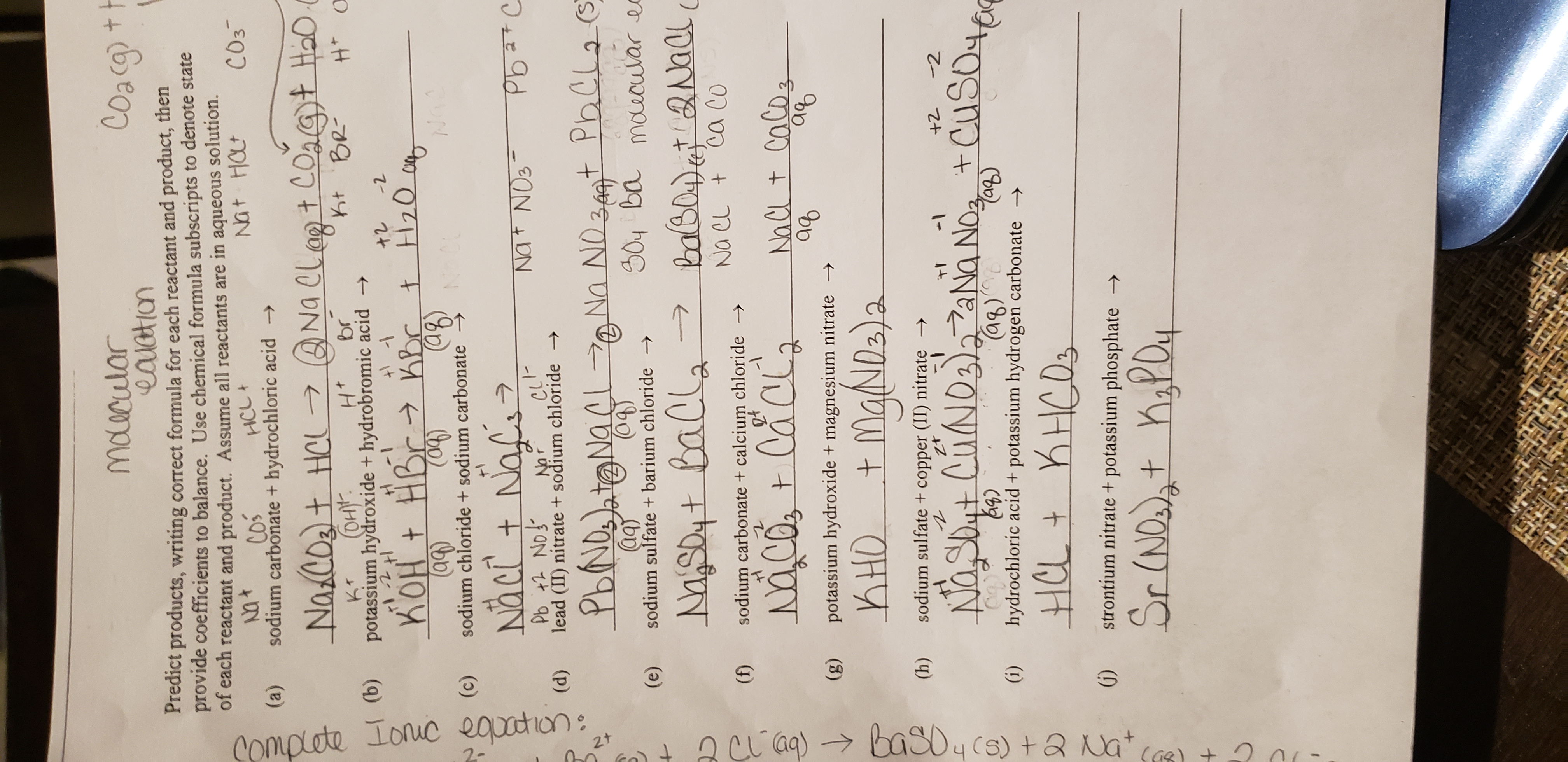# mdrulor Predict provide coefficients to balance. Use chemical formula subscripts to denote state of each reactant and product. Assume all reactants are in aqueous solution. products, writing correct formula for each reactant and product, then HCC 2 (a) sodium carbonate + hydrochloric acid -> kr (DH (b) potassium hydroxide + hydrobromic acid 1 22 C)sodium chloride + sodium carbonate (d) lead (r)nitrate +soofum oride (e) sodium sulfate + barium chloride -> MTN D7( (f) sodium carbonate+ calcium chloride -> (g) potassium hydroxide + magnesium nitrate → 2 (h) sodium sulfate+ copper (II) nitrate - T1 - +2 2 ag) (i) hydrochloric acid + potassium hydrogen carbonate 05 G) strontium nitrate + potassium phosphate

Question

Not understanding the balancing of formulas or the the complete ionic and net ionic equations.help_outlineImage Transcriptionclosemdrulor Predict provide coefficients to balance. Use chemical formula subscripts to denote state of each reactant and product. Assume all reactants are in aqueous solution. products, writing correct formula for each reactant and product, then HCC 2 (a) sodium carbonate + hydrochloric acid -> kr (DH (b) potassium hydroxide + hydrobromic acid 1 22 C)sodium chloride + sodium carbonate (d) lead (r)nitrate +soofum oride (e) sodium sulfate + barium chloride -> MTN D7( (f) sodium carbonate+ calcium chloride -> (g) potassium hydroxide + magnesium nitrate → 2 (h) sodium sulfate+ copper (II) nitrate - T1 - +2 2 ag) (i) hydrochloric acid + potassium hydrogen carbonate 05 G) strontium nitrate + potassium phosphate fullscreen

1 Rating

### Want to see the step-by-step answer?